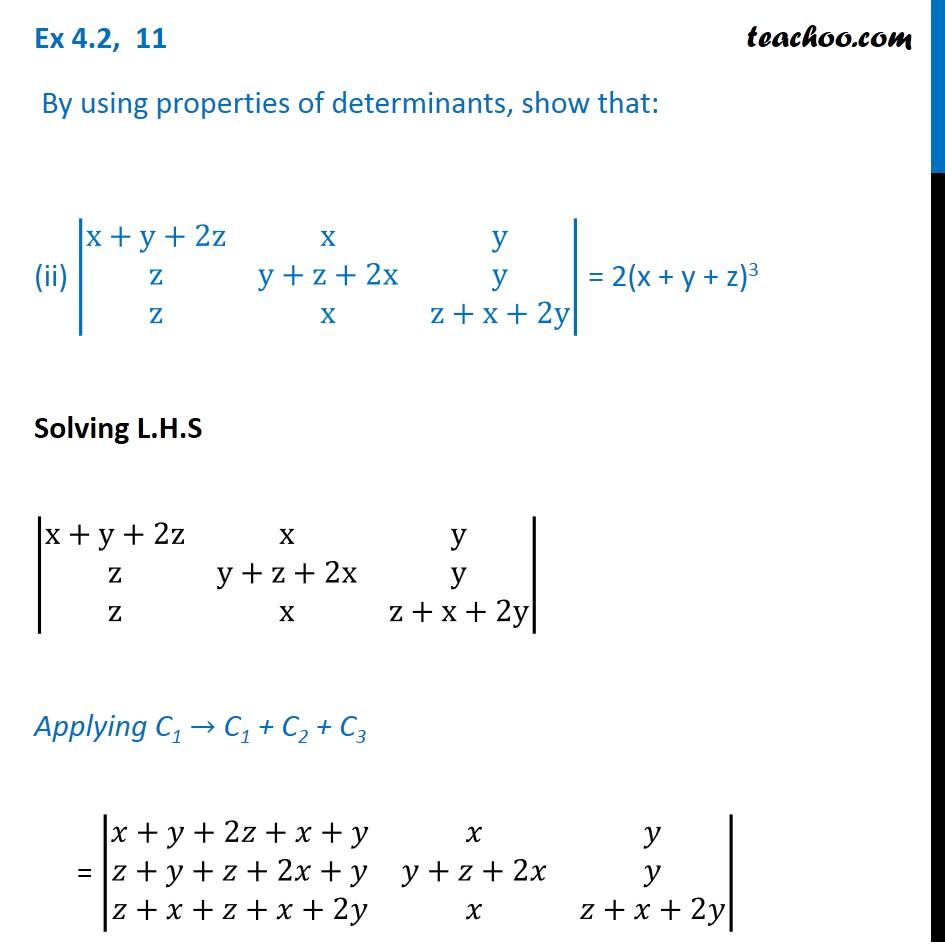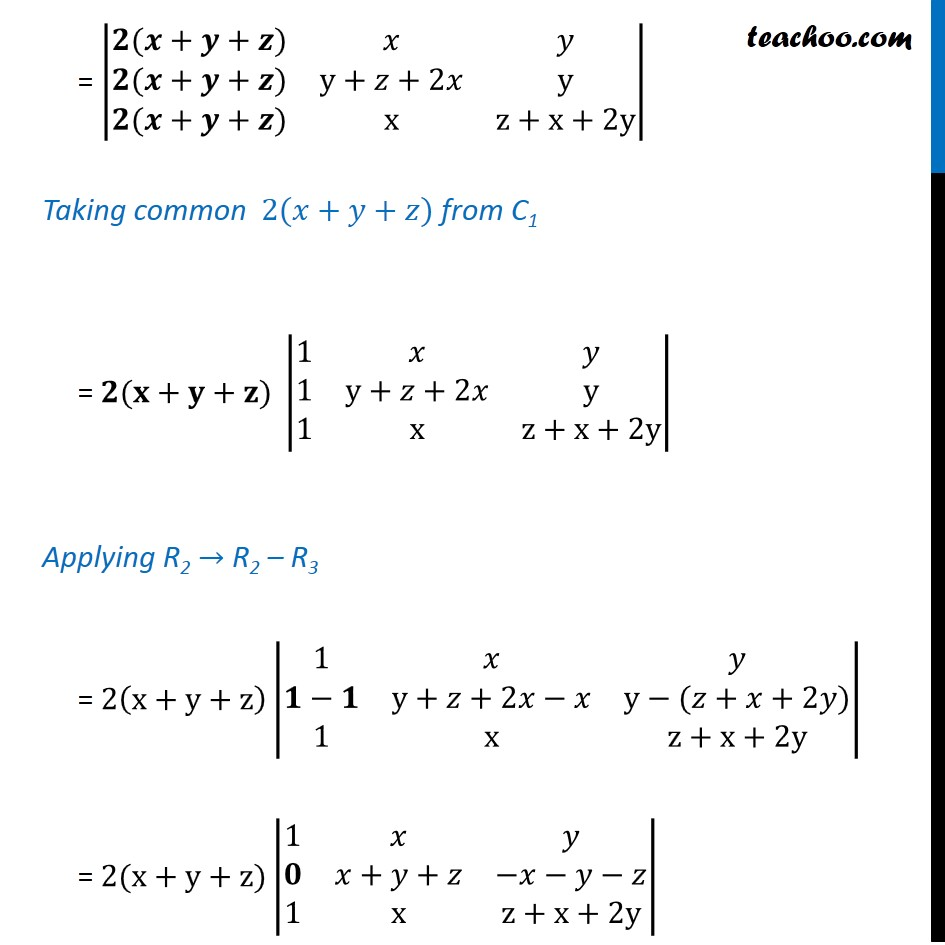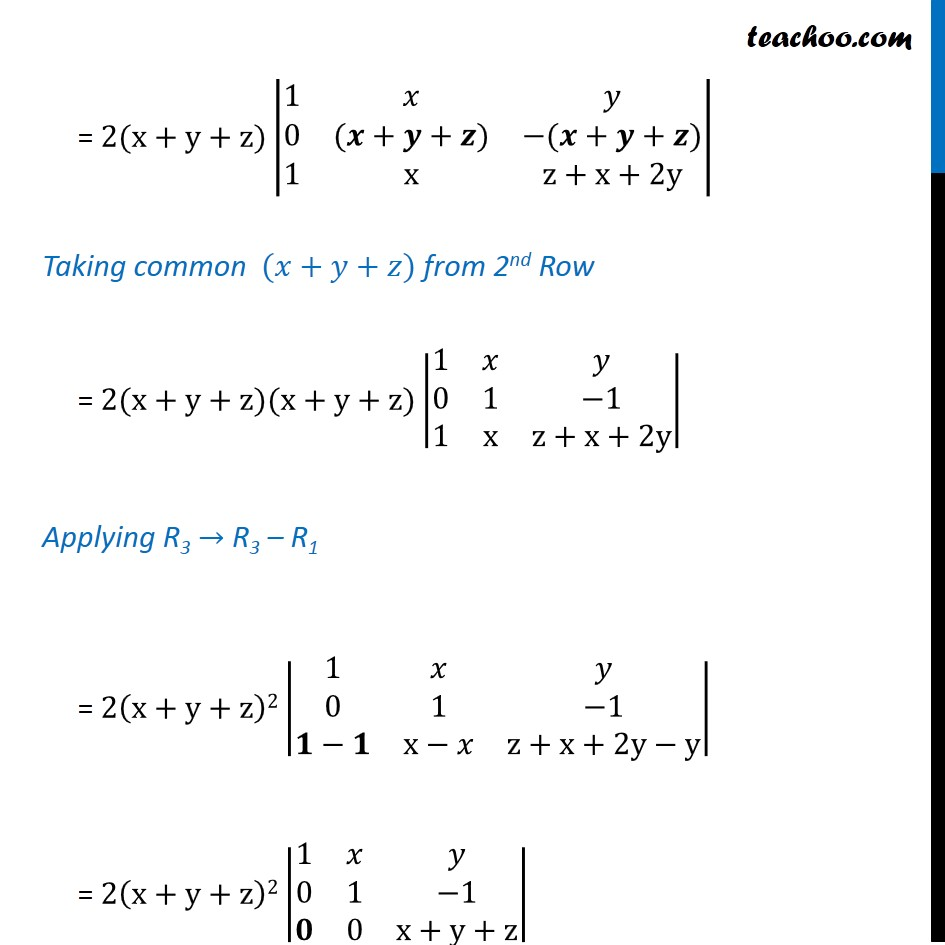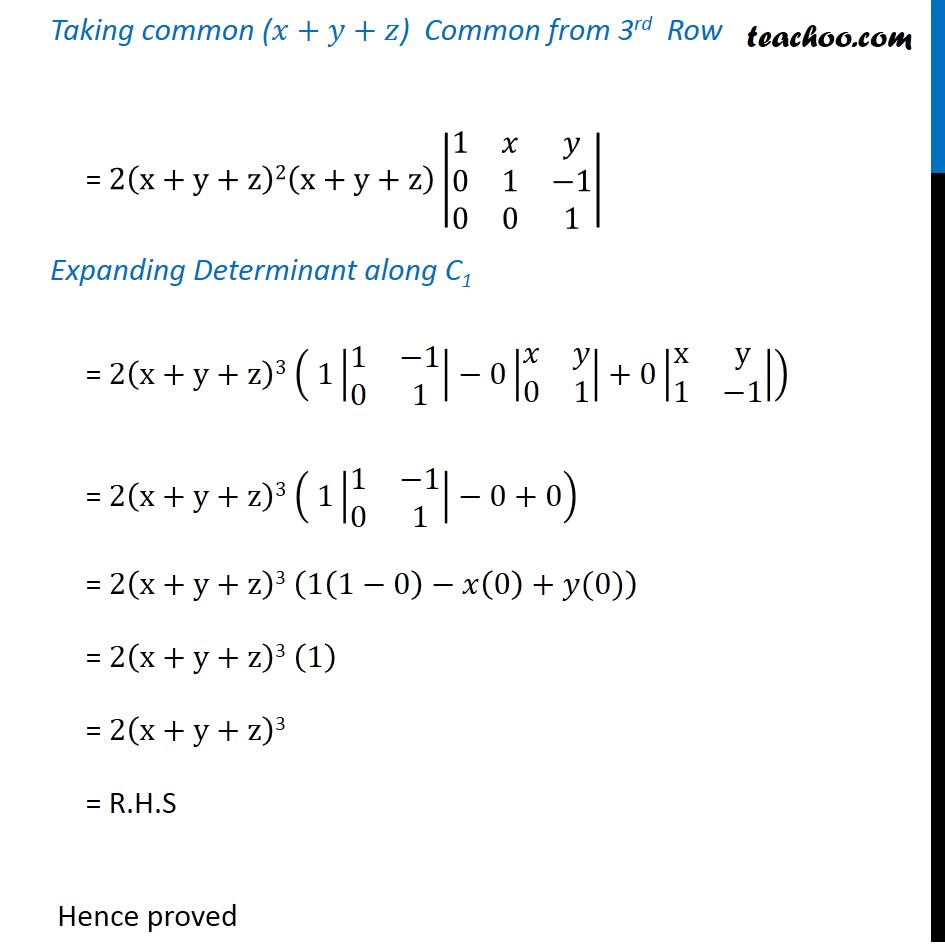Properties of Determinant

Chapter 4 Class 12 Determinants
Serial order wiseLearn in your speed, with individual attention - Teachoo Maths 1-on-1 Class

### Transcript

Question 11 By using properties of determinants, show that: (ii) |■8(x+y+2z&x&[email protected]&y+z+2x&[email protected]&x&z+x+2y)| = 2(x + y + z)3 Solving L.H.S |■8(x+y+2z&x&[email protected]&y+z+2x&[email protected]&x&z+x+2y)| Applying C1 → C1 + C2 + C3 = |■8(𝑥+𝑦+2𝑧+𝑥+𝑦&𝑥&𝑦@𝑧+𝑦+𝑧+2𝑥+𝑦&𝑦+𝑧+2𝑥&𝑦@𝑧+𝑥+𝑧+𝑥+2𝑦&𝑥&𝑧+𝑥+2𝑦)| = |■8(𝟐(𝒙+𝒚+𝒛)&𝑥&𝑦@𝟐(𝒙+𝒚+𝒛)&y+𝑧+2𝑥&y@𝟐(𝒙+𝒚+𝒛)&x&z+x+2y)| Taking common 2(𝑥+𝑦+𝑧) from C1 = 𝟐(𝐱+𝐲+𝐳) |■8(1&𝑥&𝑦@1&y+𝑧+2𝑥&[email protected]&x&z+x+2y)| Applying R2 → R2 – R3 = 2(x+y+z)|■8(1&𝑥&𝑦@𝟏−𝟏&y+𝑧+2𝑥−𝑥&y−(𝑧+𝑥+2𝑦)@1&x&z+x+2y)| = 2(x+y+z)|■8(1&𝑥&𝑦@𝟎&𝑥+𝑦+𝑧&−𝑥−𝑦−𝑧@1&x&z+x+2y)| = 2(x+y+z)|■8(1&𝑥&𝑦@0&(𝒙+𝒚+𝒛)&−(𝒙+𝒚+𝒛)@1&x&z+x+2y)| Taking common (𝑥+𝑦+𝑧) from 2nd Row = 2(x+y+z)(x+y+z)|■8(1&𝑥&𝑦@0&1&−[email protected]&x&z+x+2y)| Applying R3 → R3 – R1 = 2(x+y+z)2|■8(1&𝑥&𝑦@0&1&−1@𝟏−𝟏&x−𝑥&z+x+2y−y)| = 2(x+y+z)2|■8(1&𝑥&𝑦@0&1&−1@𝟎&0&x+y+z)| Taking common (𝑥+𝑦+𝑧) Common from 3rd Row = 2(x+y+z)2(x+y+z)|■8(1&𝑥&𝑦@0&1&−[email protected]&0&1)| Expanding Determinant along C1 = 2(x+y+z)3 ( 1|■8(1&−[email protected]&1)|−0|■8(𝑥&𝑦@0&1)|+0|■8(x&[email protected]&−1)|) = 2(x+y+z)3 ( 1|■8(1&−[email protected]&1)|−0+0) = 2(x+y+z)3 (1(1−0)−𝑥(0)+𝑦(0)) = 2(x+y+z)3 (1) = 2(x+y+z)3 = R.H.S Hence proved